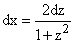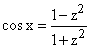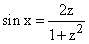## 24.3 The Substitution z = tan(x/2)

Suppose our integrand is a rational function of sin(x) and cos(x).

After the substitution z = tan(x / 2) we obtain an integrand that is a rational function of z, which can then be evaluated by partial fractions.

Theorem:

If z = tan(x / 2), then,,

andand any rational function of xdx becomes a rational function of zdz.

Proof

Example